# 深度学习基础入门篇 [9.2]：卷积之 1*1 卷积（残差网络）、2D/3D 卷积、转置卷积数学推导、应用实例

• 2023-05-22
浙江
• 本文字数：9921 字

阅读完需：约 33 分钟# 1.1*1 卷积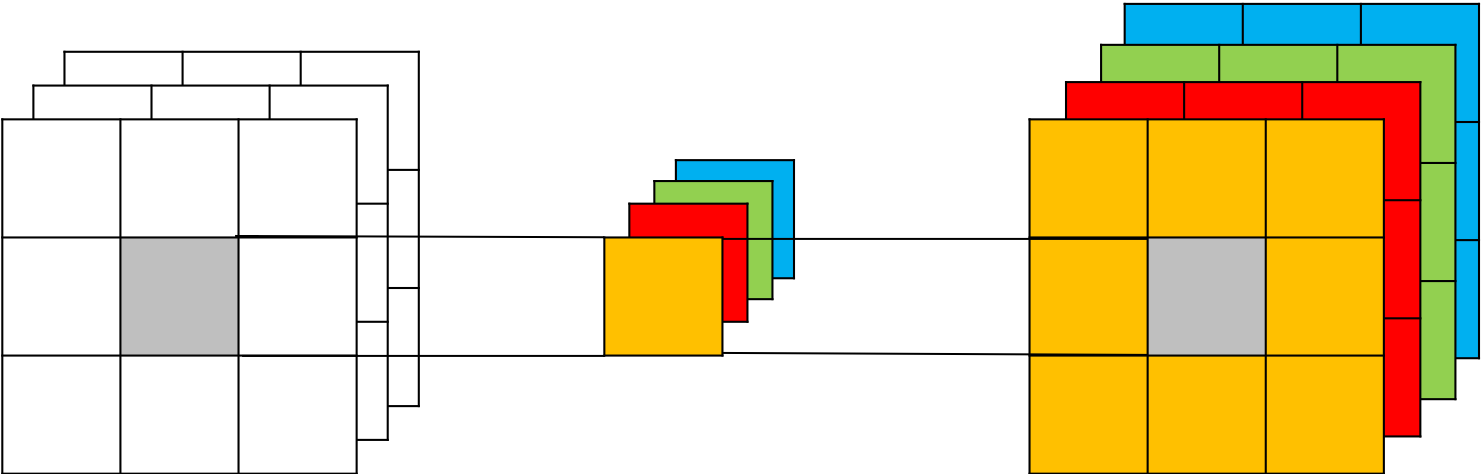• 卷积的作用

1. 实现信息的跨通道交互与整合。考虑到卷积运算的输入输出都是 3 个维度（宽、高、多通道），所以 卷积实际上就是对每个像素点，在不同的通道上进行线性组合，从而整合不同通道的信息。

2. 对卷积核通道数进行降维和升维，减少参数量。经过 卷积后的输出保留了输入数据的原有平面结构，通过调控通道数，从而完成升维或降维的作用。

3. 利用 卷积后的非线性激活函数，在保持特征图尺寸不变的前提下，大幅增加非线性

## 1.1 1*1 卷积在 GoogLeNet 中的应用

GoogLeNet 是 2014 年 ImageNet 比赛的冠军，它的主要特点是网络不仅有深度，还在横向上具有“宽度”。由于图像信息在空间尺寸上的巨大差异，如何选择合适的卷积核来提取特征就显得比较困难了。空间分布范围更广的图像信息适合用较大的卷积核来提取其特征；而空间分布范围较小的图像信息则适合用较小的卷积核来提取其特征。为了解决这个问题，GoogLeNet 提出了一种被称为 Inception 模块的方案。如 图 2 所示：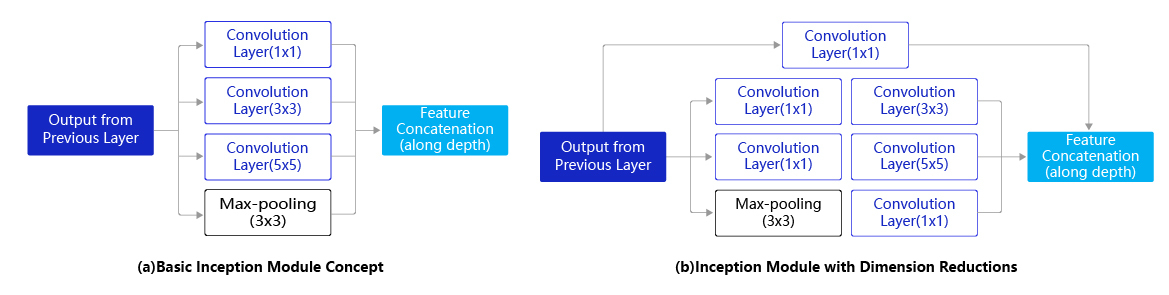Inception 模块的设计思想采用多通路(multi-path)的设计形式，每个支路使用不同大小的卷积核，最终输出特征图的通道数是每个支路输出通道数的总和。如 图 2(a) 所示，Inception 模块使用 3 个不同大小的卷积核对输入图片进行卷积操作，并附加最大池化，将这 4 个操作的输出沿着通道维度进行拼接，构成的输出特征图将会包含经过不同大小的卷积核提取出来的特征，从而达到捕捉不同尺度信息的效果。然而，这将会导致输出通道数变得很大，尤其是将多个 Inception 模块串联操作的时候，模型参数量会变得非常大。

Inception 模块的具体实现如下代码所示：

# GoogLeNet模型代码import numpy as npimport paddlefrom paddle.nn import Conv2D, MaxPool2D, AdaptiveAvgPool2D, Linear## 组网import paddle.nn.functional as F# 定义Inception块class Inception(paddle.nn.Layer):    def __init__(self, c0, c1, c2, c3, c4, **kwargs):        '''        Inception模块的实现代码，                c1,图(b)中第一条支路1x1卷积的输出通道数，数据类型是整数        c2,图(b)中第二条支路卷积的输出通道数，数据类型是tuple或list,                其中c2是1x1卷积的输出通道数，c2是3x3        c3,图(b)中第三条支路卷积的输出通道数，数据类型是tuple或list,                其中c3是1x1卷积的输出通道数，c3是3x3        c4,图(b)中第一条支路1x1卷积的输出通道数，数据类型是整数        '''        super(Inception, self).__init__()        # 依次创建Inception块每条支路上使用到的操作        self.p1_1 = Conv2D(in_channels=c0,out_channels=c1, kernel_size=1)        self.p2_1 = Conv2D(in_channels=c0,out_channels=c2, kernel_size=1)        self.p2_2 = Conv2D(in_channels=c2,out_channels=c2, kernel_size=3, padding=1)        self.p3_1 = Conv2D(in_channels=c0,out_channels=c3, kernel_size=1)        self.p3_2 = Conv2D(in_channels=c3,out_channels=c3, kernel_size=5, padding=2)        self.p4_1 = MaxPool2D(kernel_size=3, stride=1, padding=1)        self.p4_2 = Conv2D(in_channels=c0,out_channels=c4, kernel_size=1)    def forward(self, x):        # 支路1只包含一个1x1卷积        p1 = F.relu(self.p1_1(x))        # 支路2包含 1x1卷积 + 3x3卷积        p2 = F.relu(self.p2_2(F.relu(self.p2_1(x))))        # 支路3包含 1x1卷积 + 5x5卷积        p3 = F.relu(self.p3_2(F.relu(self.p3_1(x))))        # 支路4包含 最大池化和1x1卷积        p4 = F.relu(self.p4_2(self.p4_1(x)))        # 将每个支路的输出特征图拼接在一起作为最终的输出结果        return paddle.concat([p1, p2, p3, p4], axis=1)

## 1.2 1*1 卷积在 ResNet 中的应用--残差网络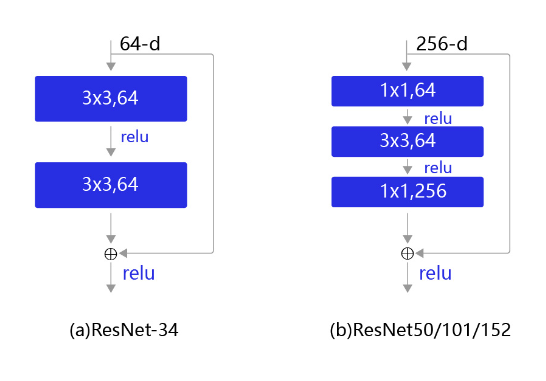# ResNet模型代码import numpy as npimport paddleimport paddle.nn as nnimport paddle.nn.functional as F# ResNet中使用了BatchNorm层，在卷积层的后面加上BatchNorm以提升数值稳定性# 定义卷积批归一化块class ConvBNLayer(paddle.nn.Layer):    def __init__(self,                 num_channels,                 num_filters,                 filter_size,                 stride=1,                 groups=1,                 act=None):               """        num_channels, 卷积层的输入通道数        num_filters, 卷积层的输出通道数        stride, 卷积层的步幅        groups, 分组卷积的组数，默认groups=1不使用分组卷积        """        super(ConvBNLayer, self).__init__()        # 创建卷积层        self._conv = nn.Conv2D(            in_channels=num_channels,            out_channels=num_filters,            kernel_size=filter_size,            stride=stride,            padding=(filter_size - 1) // 2,            groups=groups,            bias_attr=False)        # 创建BatchNorm层        self._batch_norm = paddle.nn.BatchNorm2D(num_filters)                self.act = act    def forward(self, inputs):        y = self._conv(inputs)        y = self._batch_norm(y)        if self.act == 'leaky':            y = F.leaky_relu(x=y, negative_slope=0.1)        elif self.act == 'relu':            y = F.relu(x=y)        return y# 定义残差块# 每个残差块会对输入图片做三次卷积，然后跟输入图片进行短接# 如果残差块中第三次卷积输出特征图的形状与输入不一致，则对输入图片做1x1卷积，将其输出形状调整成一致class BottleneckBlock(paddle.nn.Layer):    def __init__(self,                 num_channels,                 num_filters,                 stride,                 shortcut=True):        super(BottleneckBlock, self).__init__()        # 创建第一个卷积层 1x1        self.conv0 = ConvBNLayer(            num_channels=num_channels,            num_filters=num_filters,            filter_size=1,            act='relu')        # 创建第二个卷积层 3x3        self.conv1 = ConvBNLayer(            num_channels=num_filters,            num_filters=num_filters,            filter_size=3,            stride=stride,            act='relu')        # 创建第三个卷积 1x1，但输出通道数乘以4        self.conv2 = ConvBNLayer(            num_channels=num_filters,            num_filters=num_filters * 4,            filter_size=1,            act=None)        # 如果conv2的输出跟此残差块的输入数据形状一致，则shortcut=True        # 否则shortcut = False，添加1个1x1的卷积作用在输入数据上，使其形状变成跟conv2一致        if not shortcut:            self.short = ConvBNLayer(                num_channels=num_channels,                num_filters=num_filters * 4,                filter_size=1,                stride=stride)        self.shortcut = shortcut        self._num_channels_out = num_filters * 4    def forward(self, inputs):        y = self.conv0(inputs)        conv1 = self.conv1(y)        conv2 = self.conv2(conv1)        # 如果shortcut=True，直接将inputs跟conv2的输出相加        # 否则需要对inputs进行一次卷积，将形状调整成跟conv2输出一致        if self.shortcut:            short = inputs        else:            short = self.short(inputs)        y = paddle.add(x=short, y=conv2)        y = F.relu(y)        return y

# 2. 3D 卷积（3D Convolution）

## 2.1 3D 卷积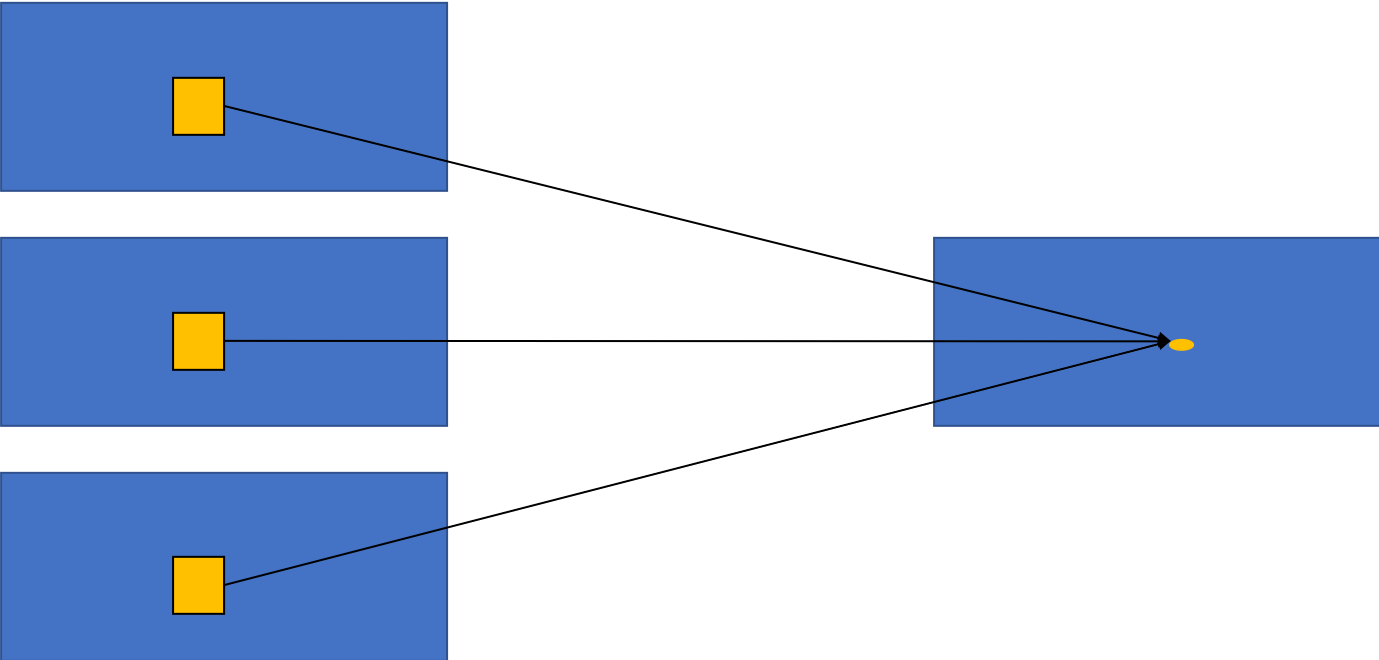2D 卷积仅仅考虑 2D 图片的空间信息，所以只适用于单张 2D 图片的视觉理解任务。在处理 3D 图像或视频时，网络的输入多了一个维度，输入由 变为了 ，其中 是通道数，为输入数据的宽度。因此，对该数据进行处理时，就需要卷积也作出相应的变换，由 2D 卷积变为 3D 卷积。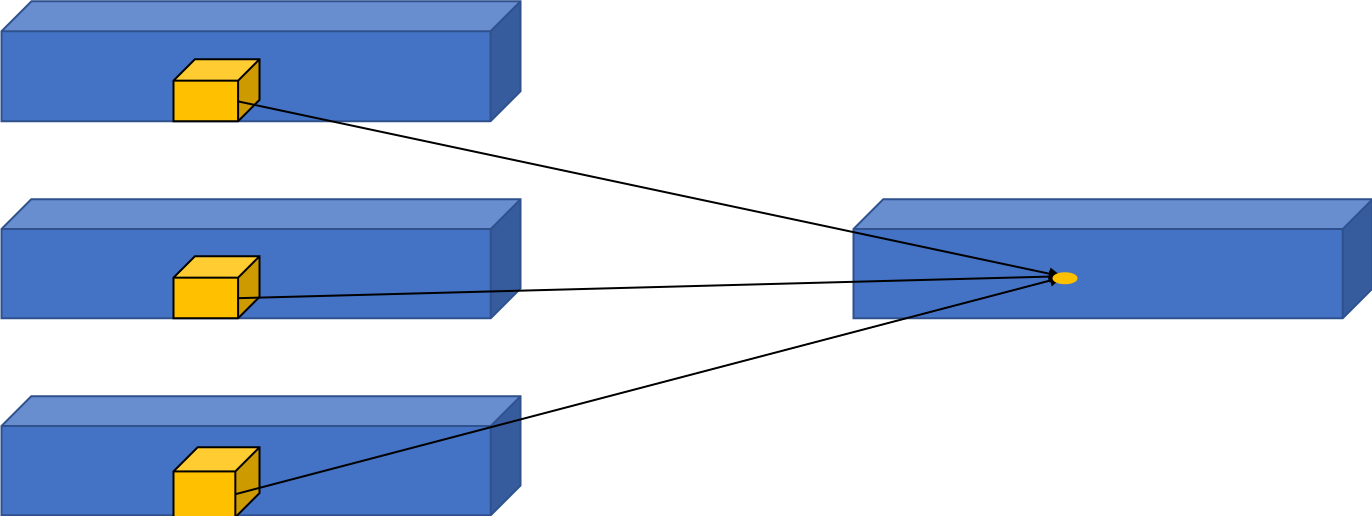## 2.2 3D 卷积应用示例

3D 卷积的主要应用就是视频理解和医疗图像领域。

3D CNN 中，使用了 3D 卷积对人体行为进行识别，网络结构如 图 3 所示。网络只有 3 个卷积层、1 个全连接层以及 2 个池化层。其中，前两个卷积层为 3D 卷积层，卷积核大小为 ，也就是说每个卷积核处理 3 个连续帧中 大小的区域。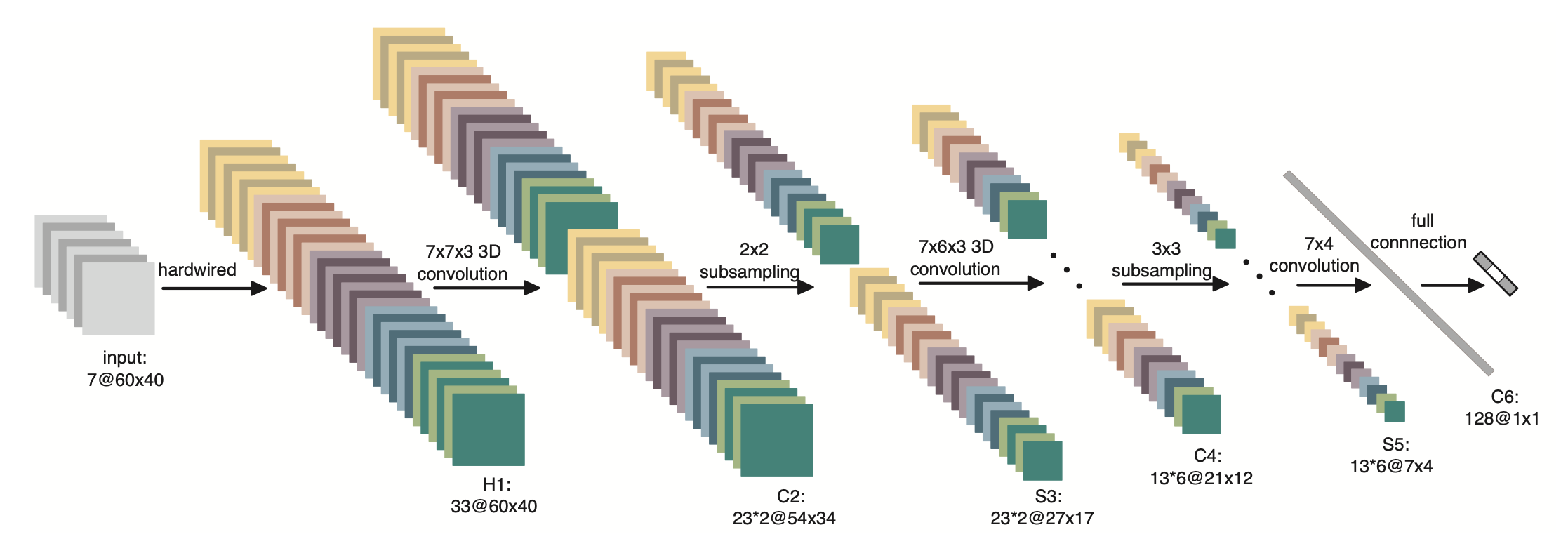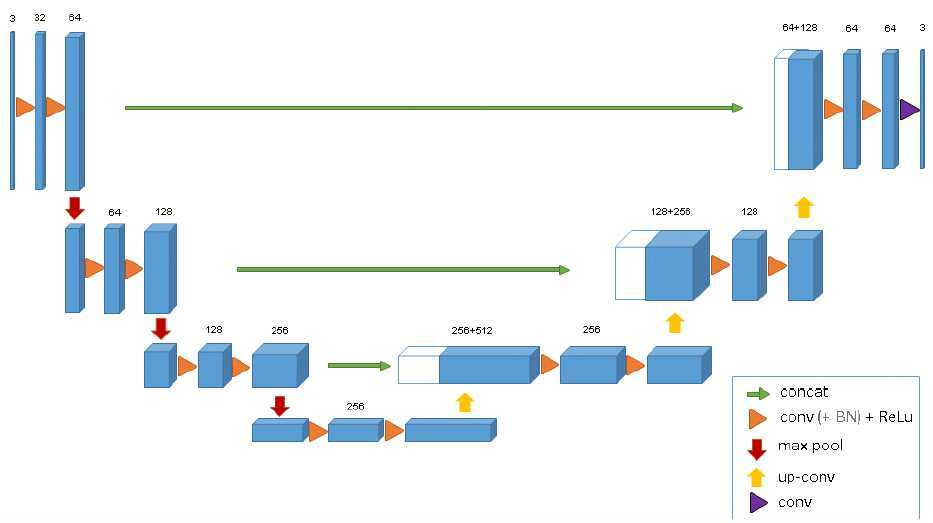# 3.转置卷积（Transpose Convolution）

## 3.1 转置卷积提出背景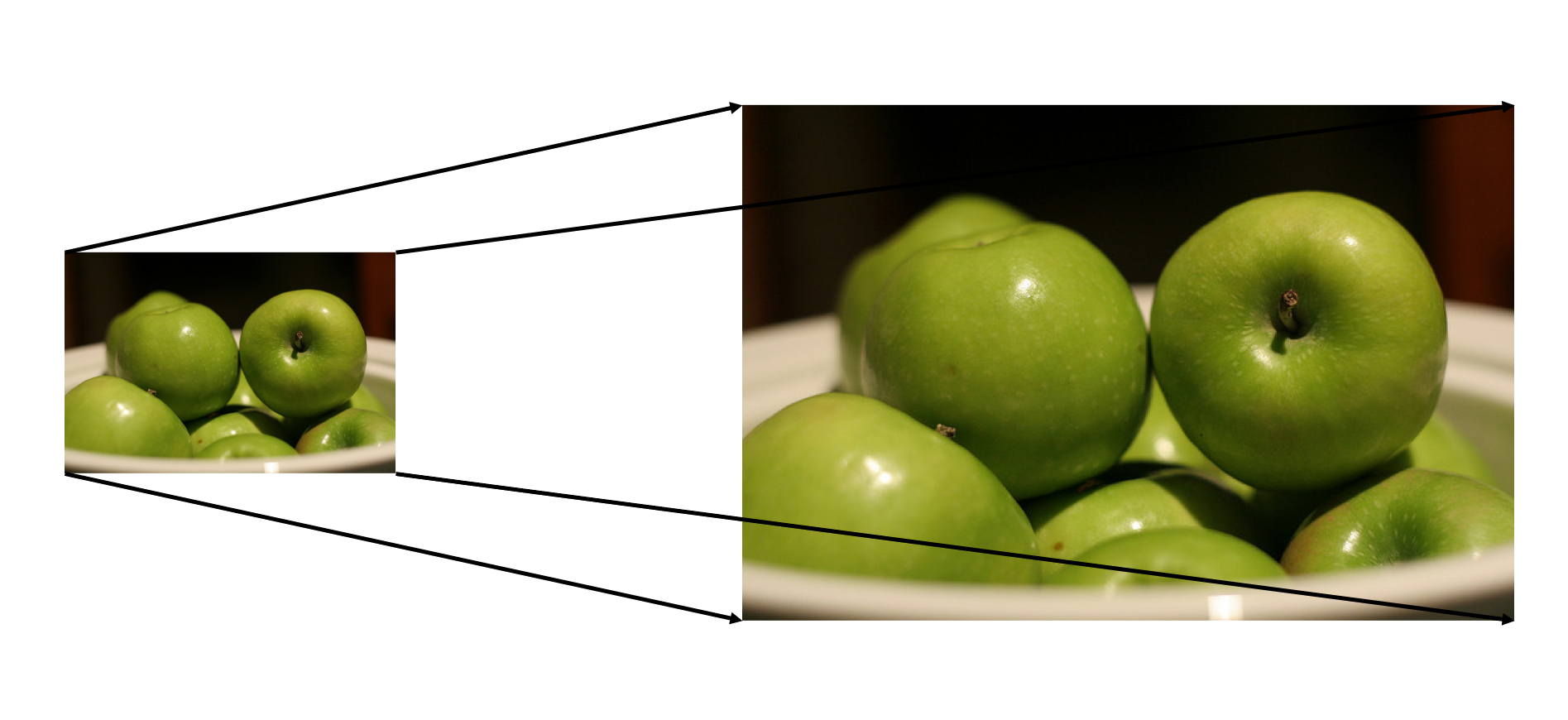## 3.2 转置卷积及其应用

• 在 DCGAN，生成器将会用随机值转变为一个全尺寸(full-size)的图片，这个时候就需要用到转置卷积。

• 在语义分割中，会使用卷积层在编码器中进行特征提取，然后在解码层中进行恢复为原先的尺寸，这样才可以对原来图像的每个像素都进行分类。这个过程同样需要用到转置卷积。经典方法如：FCN和 Unet。

• CNN 的可视化：通过转置卷积将 CNN 中得到的特征图还原到像素空间，以观察特定的特征图对哪些模式的图像敏感。

## 3.3 转置卷积与标准卷积的区别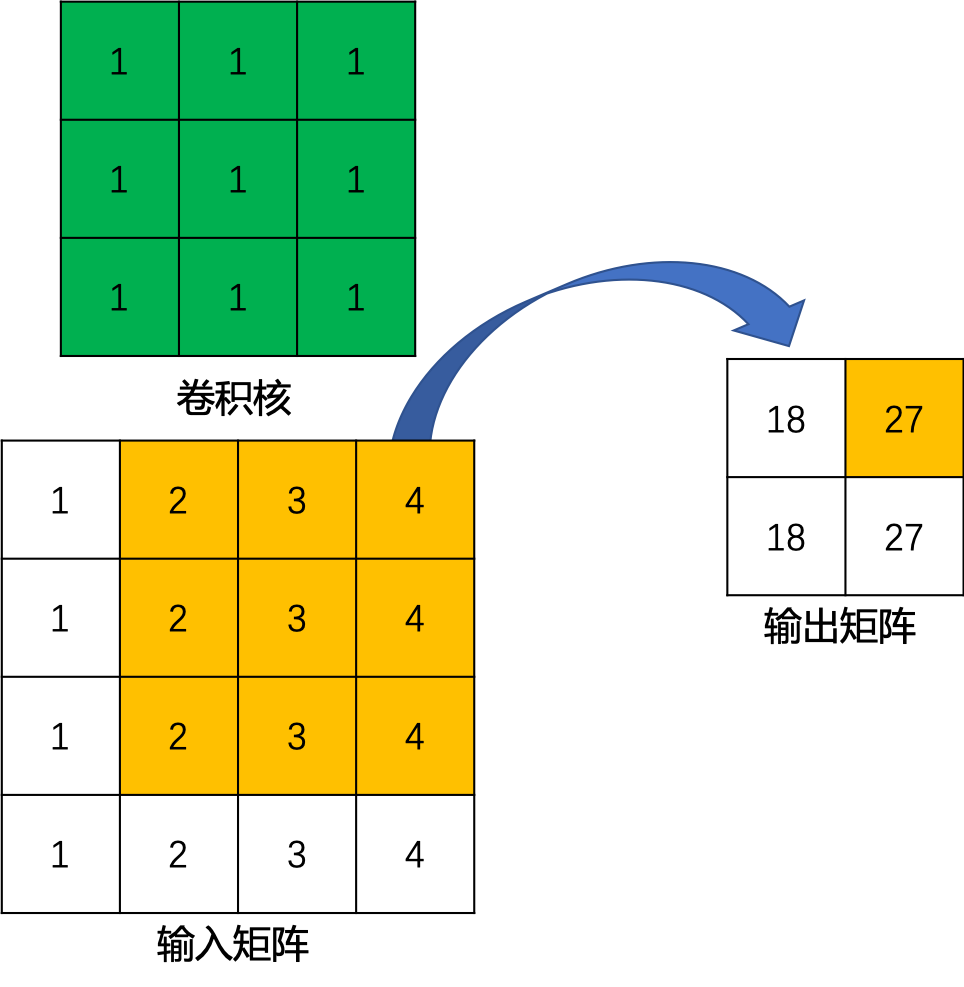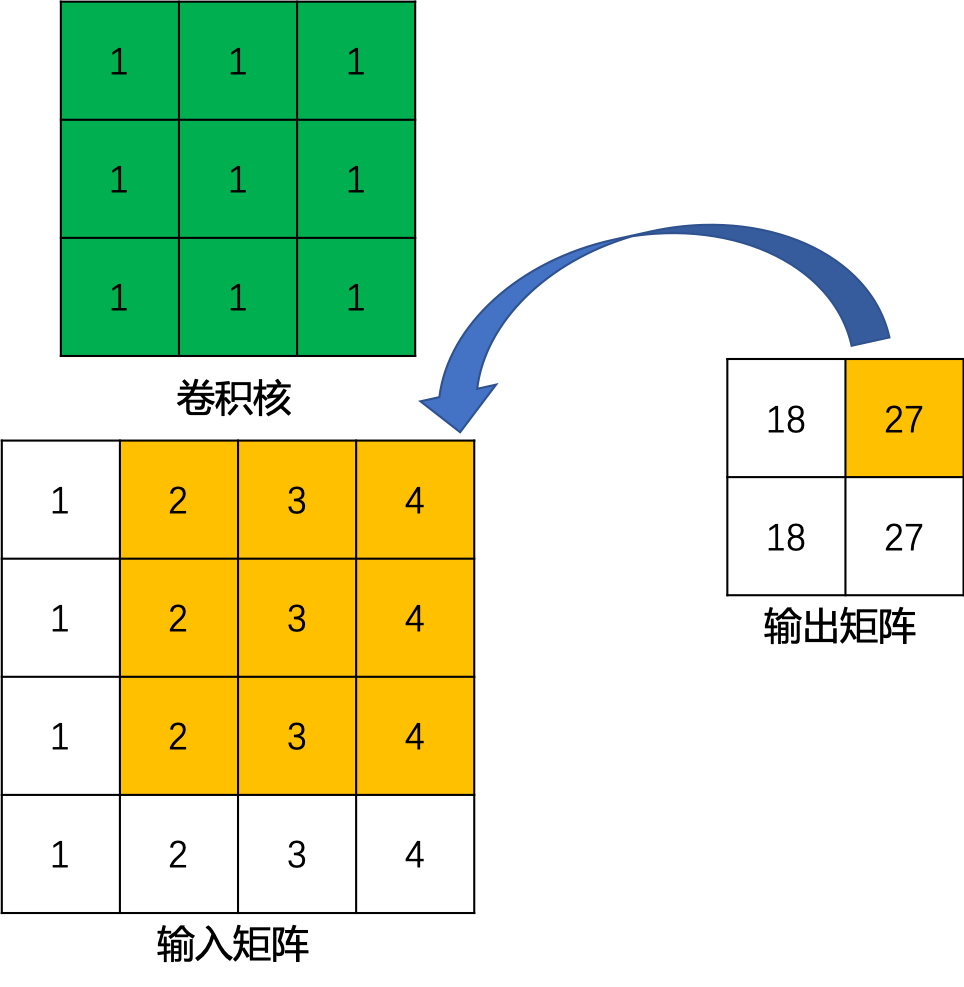## 3.4 转置卷积数学推导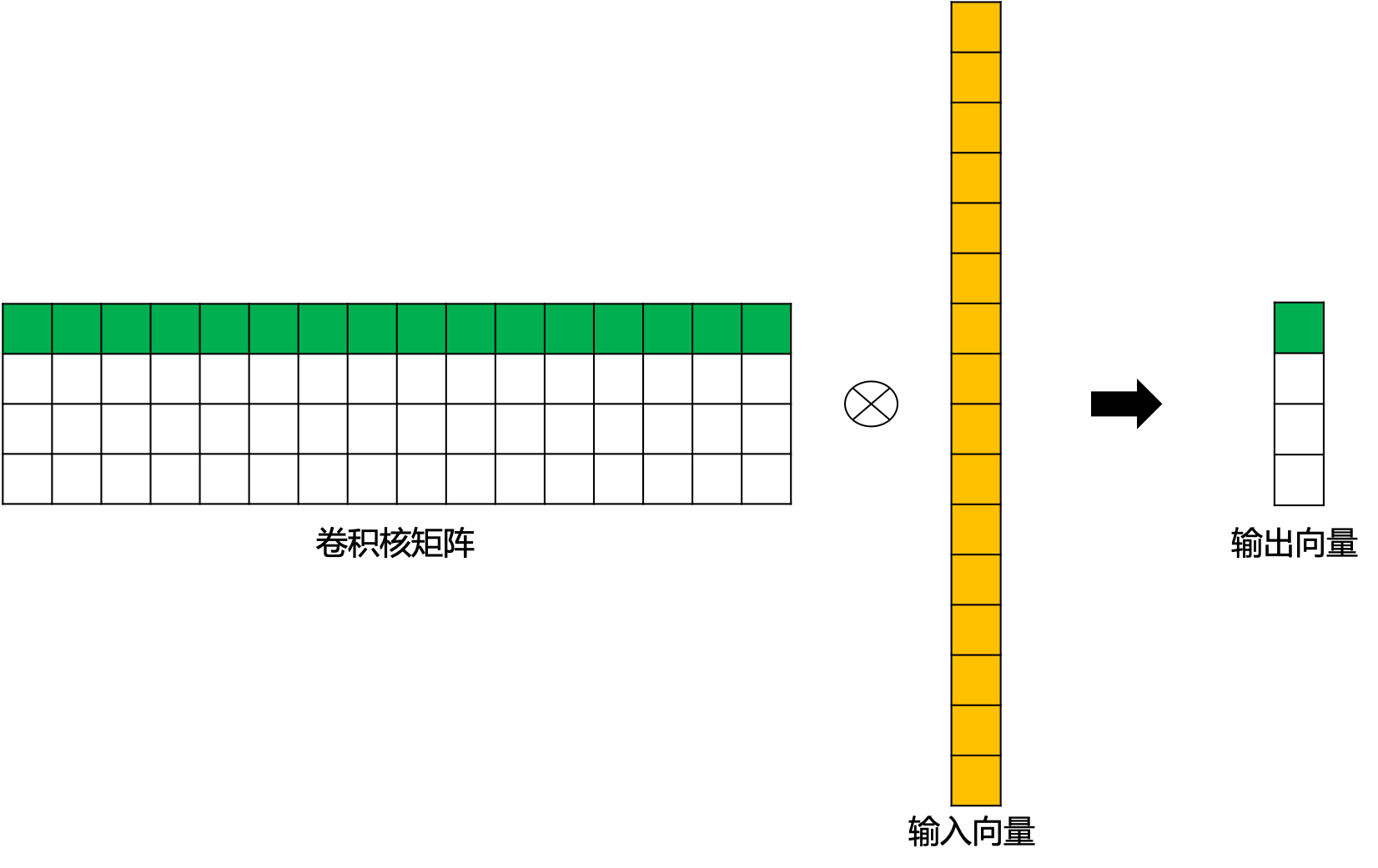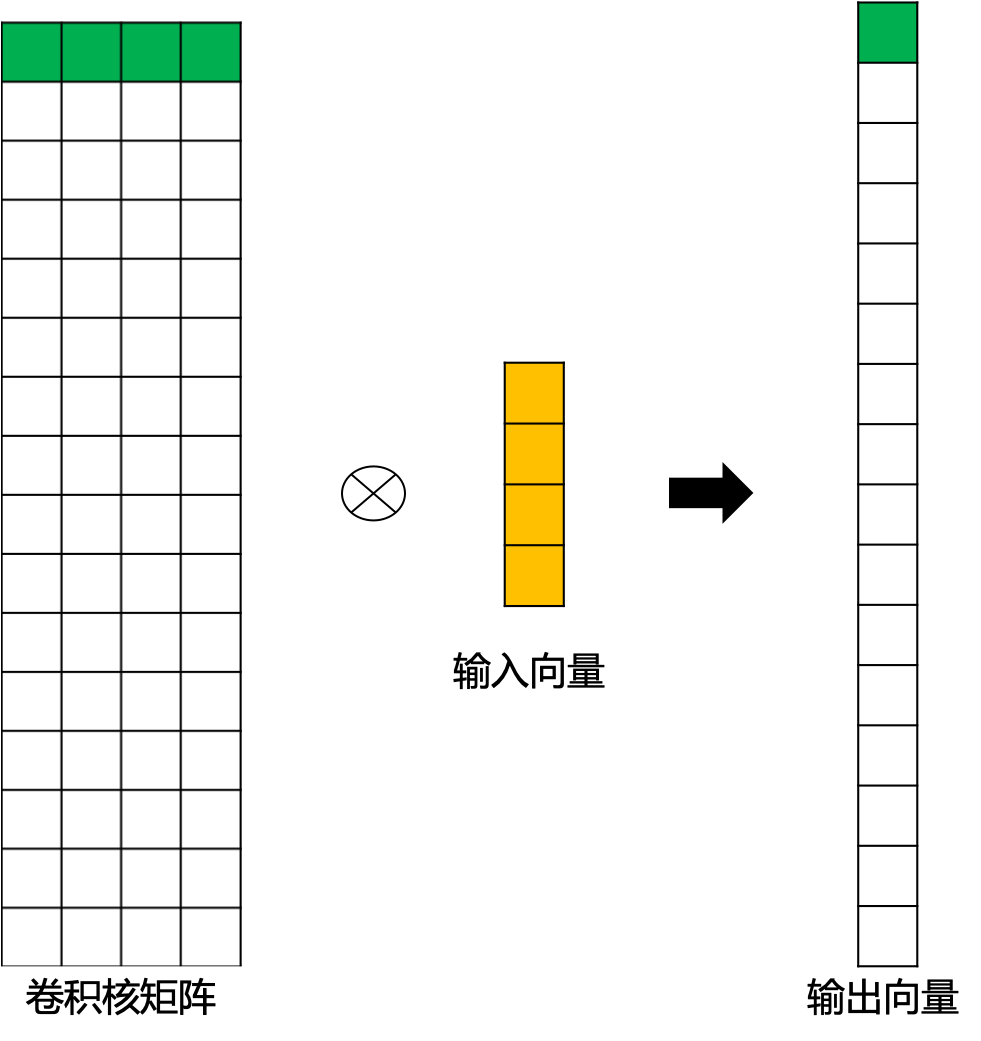## 3.5 转置卷积输出特征图尺寸

• stride=1 的转置卷积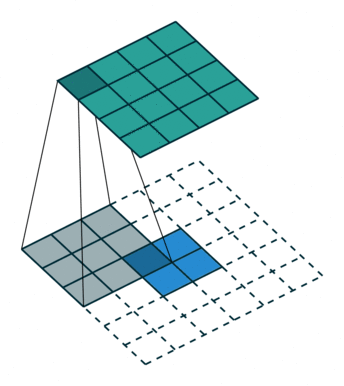• stride>1 的转置卷积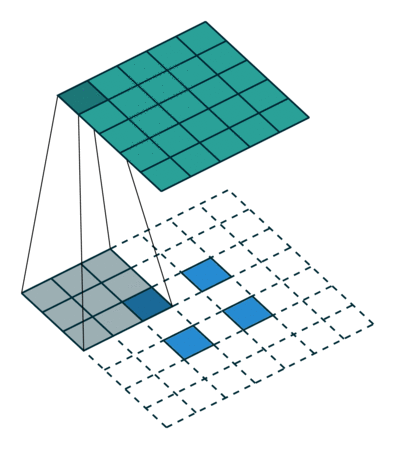## 评论